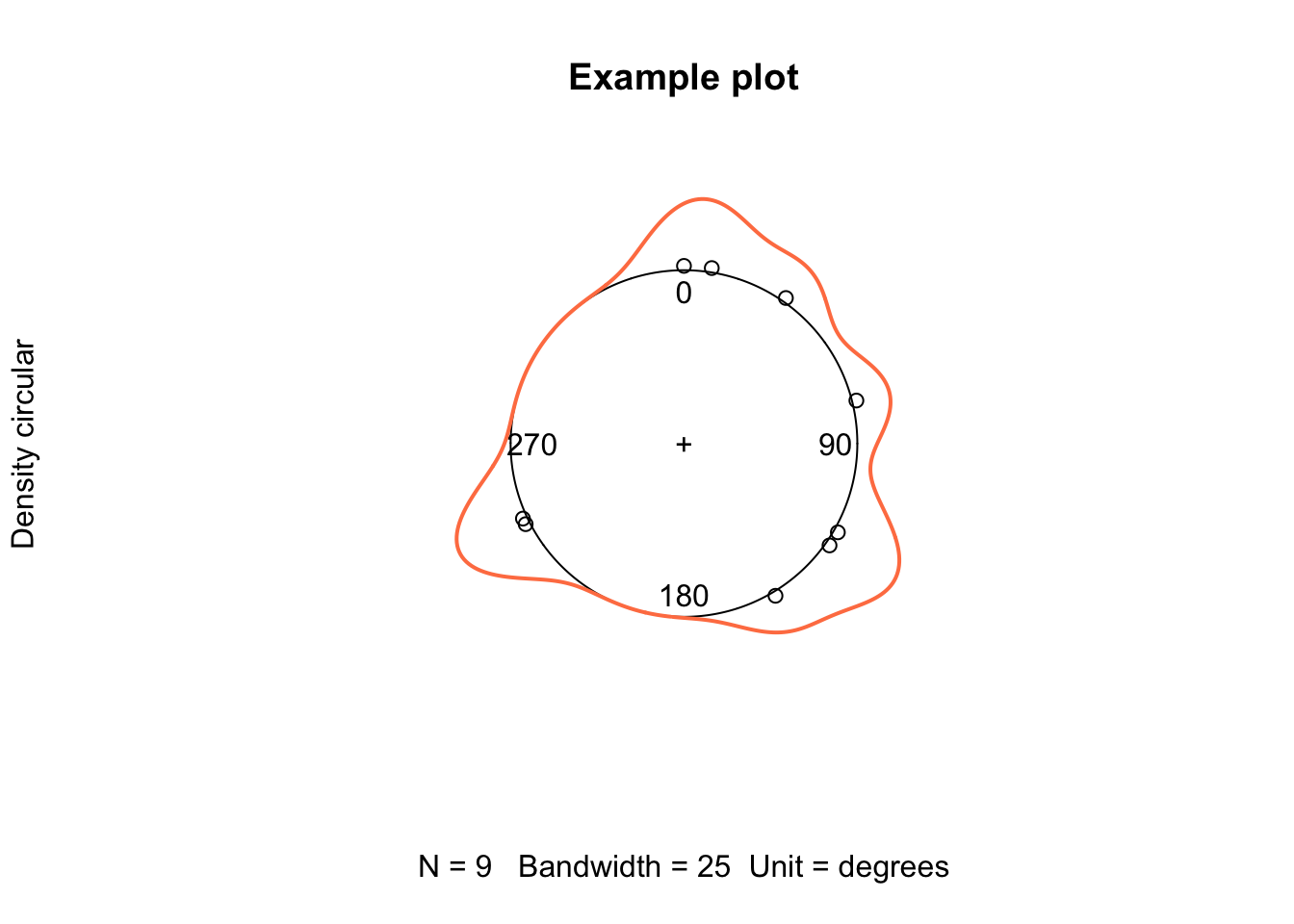### Creating Circular Distribution Graphs in R (Useful for Angular Data)

Creating Circular Distribution Graphs in R (Useful for Angular Data)

Below is an example of creating circular distribution/density plots. It could be useful while exploring angular data, where, for example, the value of 360 degrees is equal to the value of 0 degree, hence a circular representation is more appropriate. In the computational biology context, this type of graphs could be useful to explore dihedral angle distribution in, say, a set of protein or nucleic acids structures, or, with some modifications, to explore distribution of a certain parameter along a circular genome.

We need the R library circular, so if that is not installed, install from within R using the command below:

install.packages("circular")

Let us create an example dataset:

data.vector <- c(120, 125, 149, 35, 360, 245, 9, 76, 243)

If our dataset contains negative angles, for instance, angles in the $$[-180,180)$$ range, we can change the representation into the $$[0,360)$$ range via the following line:

data[which(data < 0)] <- 360 + data[which(data < 0)]

Now, we can write a small function, plotcircle, to read a vector of angular values and produce a circular density plot visualising the distribution of those values:

plotcircle <- function(data, bw = 25, ...) {

library(circular)

data <- suppressWarnings(as.circular(data, control.circular = list(type = "angles",
zero = pi/2, units = "degrees", rotation = "clock")))

denscirc <- density.circular(as.circular(data), bw = bw)
plot(denscirc, points.plot = TRUE, ...)
}

And, here is the example visualisation of the created data.vector dataset:

plotcircle(data = data.vector, bw = 25, col = "coral", lwd = 2, xlim = c(-1.5,
1.5), ylim = c(-1.5, 1.5), main = "Example plot")
##
## Attaching package: 'circular'
##
## The following objects are masked from 'package:stats':
##
##     sd, varYou can explore the documentation of the library circular, to see what modifications can be done to the above code, specifically to the as.circular and density.circular calls, to suite your specific needs.